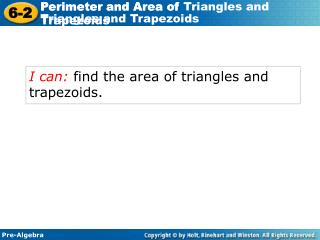# I can: find the area of triangles and trapezoids. - PowerPoint PPT PresentationDownload PresentationI can: find the area of triangles and trapezoids.

I can: find the area of triangles and trapezoids.
Download Presentation## I can: find the area of triangles and trapezoids.

- - - - - - - - - - - - - - - - - - - - - - - - - - - E N D - - - - - - - - - - - - - - - - - - - - - - - - - - -
##### Presentation Transcript

1. Perimeter and Area of Triangles and Trapezoids I can: find the area of triangles and trapezoids.

2. The figures show a fractal called the Koch snowflake. It is constructed by first drawing an equilateral triangle. Then triangles with sides one-third the length of the original sides are added to the middle of each side. The second step is then repeated over and over again.

3. The area and perimeter of each figure is larger than that of the one before it. However, the area of any figure is never greater than the area of the box, while the perimeters increase without bound. To find the area and perimeter of each figure, you must be able to find the area of a triangle.

4. 11 8 6 17 Additional Example 1: Finding the Perimeter of Triangles and Trapezoids Find the perimeter of each figure. 7 A. Add all sides. 4 P = 4 + 7 + 10 10 = 21 units Add all sides. B. P = 8 + 11 + 6 + 17 = 42 units

5. Try This: Example 1 Find the perimeter of each figure. Add all sides. 9 6 P = 9 + 6 + 11 A. = 26 units 11 22 Add all sides. B. 6 P = 6 + 22 + 5 + 16 5 = 49 units 16

6. A triangle or trapezoid can be thought of as half of a parallelogram.

7. A = (8)(4) = 16 units2 1 2 Triangle: The area A of a triangle is one-half the base length b times the height h.

8. A = (2)(3 + 7) = 10 units2 A = (h)(b1 + b2) 1 2 1 2 Trapezoid: The area of a trapezoid is one-half the height h times the sum of the base lengths b1and b2.

9. y 1 2 A =bh 1 2 = • 6 • 3 x Additional Example 2A: Finding the Area of Triangles and Trapezoids Graph and find the area of the figure with the given vertices. A. (–2, 2), (4, 2), (0, 5) Area of a triangle (0, 5) Substitute for b and h. 3 (–2, 2) (4, 2) 6 = 9 units2

10. 1 2 A =h(b1 + b2) 1 2 = • 6(8 + 4) Additional Example 2B: Finding the Area of Triangles and Trapezoids Graph and find the area of the figure with the given vertices. B. (–1, –2), (5, –2), (5, 2), (–1, 6) y (–1, 6) Area of a trapezoid (5, 2) Substitute for h, b1, and b2. 8 4 x 6 (–1, –2) (5, –2) = 36 units2

11. y 1 2 A =bh 1 2 = • 8 • 6 x Try This: Example 2A Graph and find the area of the figure with the given vertices. A. (–1, 1), (1, 7), (7, 1) Area of a triangle (1, 7) Substitute for b and h. 6 (7, 1) (–1, 1) = 24 units2 8

12. 3 1 2 A =h(b1 + b2) 6 1 2 7 = • 6(7 + 3) Try This: Example 2B Graph and find the area of the figure with the given vertices. B. (–2, –1), (0, 5), (3, 5), (5, –1) y Area of a trapezoid (3, 5) (0, 5) Substitute for h, b1, and b2. x (–2, –1) (5, –1) = 30 units2

13. Lesson Quiz Use the figure to find the following measurements. 1. The perimeter of the triangle 36 cm 44 cm 2. The perimeter of the trapezoid 64 cm 3. The perimeter of the combined figure 4. The area of the triangle 54 cm2 104 cm2 5. The area of the trapezoid